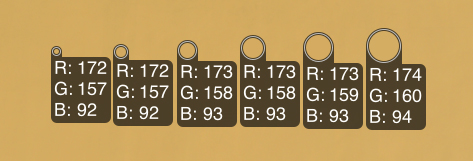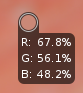# Number of pixels read by Lockable Color Picker

Hi everyone, I tried to search but without success. I would like to know how many pixels read the different sizes that can be chosen with tool “Lockable Color Picker”.Thanks

Interesting question. I believe the size is fixed at 8, but probably @heckflosse can confirm. See here in the code: https://github.com/Beep6581/RawTherapee/blob/f0720ff34c0e905ce819712068b5dfd8a8bd306a/rtgui/editcallbacks.cc#L274-L277

But I also think there is a bug, because the different optical sizes are clearly an illusion. They also seem to behave wrongly when you zoom the image.

… eight pixels? Strange. Wouldn’t it be more logical to start a reading of 1 pixel, then a 3x3 square (9 pixels) and multiples? Photoshop for example uses this pixel pattern.
In the code at the link you posted, at the bottom row there is an “8”, but I’m not an expert on codes and I don’t know what it can refer to.

The number of pixels are the mumber of pixels which fit into a circle of diameter 5, 10, 15, 20, 25 or 30

1 Like

… thanks for your reply heckflosse. I am puzzled by the number of pixels read. Ten pixels, for example, how are they arranged? On one line? Or on two rows of five pixels?

example for 10

``````x x x x x x x x x x
x x x x x x x x x x
x x x x x x x x x x
x x x x x x x x x x
x x x x x x x x x x
x x x x x x x x x x
x x x x x x x x x x
x x x x x x x x x x
x x x x x x x x x x
x x x x x x x x x x
``````

draw a circle on this square and look at the pixels inside the circle.

Edit: that’s roughly how it works. For details better ask @Hombre

… if this is the grid but the area is a circle, it means that several pixels remain outside the reading area. At this point the reading value to consider is the smallest one, at least according to my workflow habits.

Maybe this was a bit unlcear. I meant: Draw a circle inside the square

… I understood what you wrote in the first answer. But what I don’t understand is how RawTherapee can read only a portion of the peripheral pixels (left in the image). While the method that Photoshop uses is to read a square grid that reads the entire pixel.

Well, the colour picker has a circle shapeIt would be confusing if the colour picker would read pixels which are completely outside the circle.
If a pixel is inside the circle (even partly), the picker will read it

Edit: in your example it does not read the 3 corner pixels for each corner.

FYI, I have made an issue to improve the visual feedback on the sampling region: https://github.com/Beep6581/RawTherapee/issues/5767

2 Likes

…thanks…

@carlos1960

I don’t really understand the question here : it is obvious, as represented by the circle, that it read’s what’s inside the circle.

So why a circle ? Because in most case, you can read more values by using a circle than a square, which corners will annoy you (again, in most case).

Also, as the documentation explain, the circle can be of different size, but each size is fixed on screen, i.e. if you zoom out, the result will include more samples because of the resize algorithm. So it’s really zoom dependent.

And for the 5px minimum diameter, I didn’t used lower values because local variability are of no practical use IMHO, and also because the pointer already shows the pixel color, though not lockable.

[…] can read only a portion of the peripheral pixels

My english is probably not good enough, but I don’t understand what you mean by peripheral here